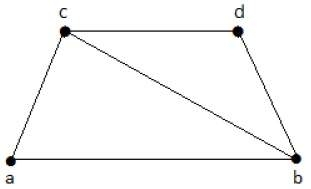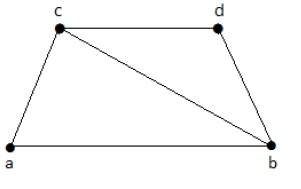# Vertex Covering

A covering graph is a subgraph that contains either all the vertices or all the edges corresponding to some other graph. A subgraph that contains all the vertices is called a line/edge covering. A subgraph that contains all the edges is called a vertex covering.

Let 'G' = (V, E) be a graph. A subset K of V is called a vertex covering of 'G', if every edge of 'G' is incident with or covered by a vertex in 'K'.

## Example

Take a look at the following graph −The subgraphs that can be derived from the above graph are as follows −

K1 = {b, c}
K2 = {a, b, c}
K3 = {b, c, d}
K4 = {a, d}

Here, K1, K2, and K3 have vertex covering, whereas K4 does not have any vertex covering as it does not cover the edge {bc}.

## Minimal Vertex Covering

A vertex 'K' of graph 'G' is said to be minimal vertex covering if no vertex can be deleted from 'K'.

## Example

In the above graph, the subgraphs having vertex covering are as follows −

K1 = {b, c}

K2 = {a, b, c}

K3 = {b, c, d}

Here, K1 and K2 are minimal vertex coverings, whereas in K3, vertex 'd' can be deleted.

## Minimum Vertex Covering

It is also known as the smallest minimal vertex covering. A minimal vertex covering of graph 'G' with a minimum number of vertices is called the minimum vertex covering.

The number of vertices in a minimum vertex covering of 'G' is called the vertex covering a number of G (α2).

## Example

In the following graph, the subgraphs having vertex covering are as follows −

K1 = {b, c}

K2 = {a, b, c}

K3 = {b, c, d}Here, K1 is a minimum vertex cover of G, as it has only two vertices. α2 = 2.